# An introduction to repeatability estimation with rptR

#### 2019-03-06

The concept of ‘repeatability’ relates to the way of quantifying the reliability of measurements. In a wider context, however, the repeatability offers insights into the components contributing to variability in the data. The repeatability describes the relative partitioning of variance into within-group and between-group sources of variance and is more generally referred to as the intra-class correlation (ICC). The hierarchical decomposition of variances has become the focus of several disciplines. In the context of behavioral research, for example, repeatability is often used to quantify stable individual differences and thus became a fundamental quantity of interest in the field of animal personality research.

A very powerful tool for estimating repeatabilities is the mixed effects model framework. Random-effect predictors are used to estimate variances at different hierarchical levels. In the simplest case of a design where repeated measures (e.g. quantifications of behavior) were taken from the same features (e.g. individuals), the repeatability $$R$$ is calculated as the variance among group means (group-level variance $$V_G$$) over the sum of group-level and data-level (residual) variance $$V_R$$: $R = {V_G}/{(V_G+V_R)}$

While it is relatively easy to extract point estimates for $$V_G$$ and $$V_R$$ from standard software packages, it is considerably more difficult to quantify the uncertainty in estimated $$R$$ values. The rptR package offers easy-to-use functions for estimating $$R$$ and its uncertainty. The package includes estimates of the repeatability (and also raw variances and marginal $$R^2$$; sensu Nakagawa and Schielzeth 2013) for Gaussian traits, binomial and Poisson-distributed traits. The theory for for the quantification of the repeatability for non-Gaussian traits is reviewed in Nakagawa & Schielzeth (2010).

The functions build heavily on functions for fitting mixed models, in particular on lmer from the lme4 package (Bates et al. 2015). Mixed-effect models are fitted within the function call and fitted model objects are returned as part of the class rpt return object. Uncertainty is quantified via parametric bootstrapping. In a nutshell, parametric bootstrapping works by (i) fitting the model, (ii) simulating new data based on the estimators of the original model while adhering to the original sampling design, (iii) refitting the model to the simulated data and storage of the repeatability of the model fit. Repeating steps (ii) to (iii) a large number of times (e.g. 1000 times) yields the sampling variance give the data structure under the assumption that the model is valid.

## Basic usage

As usual, the CRAN-version of the package can be installed via a call to:

install.packages("rptR")

Since the package is developed on Github (www.github.com), there is likely to be a developmental version that implements new features prior to release on CRAN. This current stable version on Github can be installed via a call to:

install.packages("devtools")
devtools::install_github("mastoffel/rptR", build_vignettes = TRUE)

After one of those two steps, the package can be loaded by

library(rptR)

The citation can be found with

citation("rptR")

The central wrapper function for estimating the repeatability is rpt. The argument datatype decides whether the call is forwarded to the specialized functions rptGaussian for Gaussian traits, rptBinary for binary (0/1) outcomes, rptProportion for proportion data represented as joint vectors (or matrix) of successes and failures and rptPoisson for Poisson-distributed count data. The functions return S3 class rpt objects that can be viewed via the str function. The generic functions summary, print and plot are available for a more convenient display of the results. In the following we walk through the features of rptR starting with Gaussian models for introducing the basic plots and outputs, before addressing the specifics of count and binary trait analyses.

## A toy dataset

As an example dataset we will use a simulated toy dataset that was introduced for a different purpose (Nakagawa & Schielzeth 2013). It offers a balanced dataset with rather simple structure, sizable effects and decent sample size, just right to demonstrate some features of rptR. Sufficient sample size is required in particular for the non-Gaussian traits, because those tend to be more computationally demanding and less rich in information per data point than simple Gaussian traits.

In brief, the imaginary sampling design of the simulated dataset is as follows. Beetle larvae were sampled from 12 populations (Population) with samples taken from two discrete microhabitats at each location (Habitat). Samples were split in equal proportions and raised in two dietary treatments (Treatment). Beetles were sexed at the pupal stage (Sex) and pupae were kept in single-sex containers (Container). Phenotypes (BodyL, Egg and Color) were measured at the imaginal stage and are introduced below in the separate sections.

## Repeatability for Gaussian data

As an example for Gaussian data, we will analyse body length (BodyL). To start with, we will analyse the repeatability at the level of the population that might arise through genetic differentiation and/or early environmental influences prior to sampling.

data(BeetlesBody)
str(BeetlesBody)
## 'data.frame':    960 obs. of  6 variables:
##  $Population: int 1 1 1 1 1 1 1 1 1 1 ... ##$ Container : int  1 1 1 1 1 1 1 1 2 2 ...
##  $Sex : Factor w/ 2 levels "Female","Male": 1 1 1 1 1 1 1 1 2 2 ... ##$ Habitat   : Factor w/ 2 levels "A","B": 1 1 1 1 2 2 2 2 1 1 ...
##  $Treatment : Factor w/ 2 levels "Cont","Exp": 1 2 1 2 1 2 1 2 1 2 ... ##$ BodyL     : num  10.8 11.2 11.6 15.6 12.4 ...
hist(BeetlesBody$BodyL)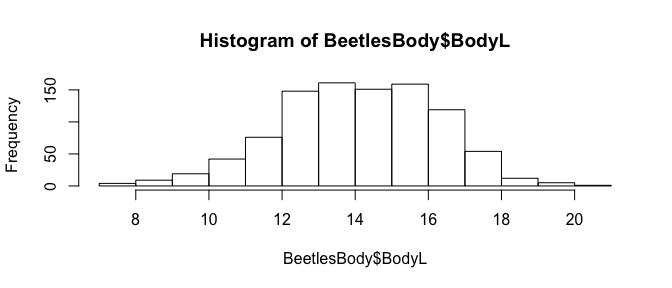table(BeetlesBody$Population)
##
##  1  2  3  4  5  6  7  8  9 10 11 12
## 80 80 80 80 80 80 80 80 80 80 80 80

We analyse the data using the rpt function with the argument datatype = "Gaussian". It is possible to call rptGaussian directly, while omitting the datatype argument. As parametric bootstrapping and permutation can be slow, we recommend starting with shutting bootstrapping and permutation off by setting nboot = 0 and npermut = 0. Note that the formula argument uses the same syntax as lmer from the lme4 packages, because the formula-call is directly forwarded to lmer. Also note that grname has to be specified with quotation marks and that the term has to match exactly a random effect as it is used in the formula argument. The data argument is mandatory and is specified without quotes.

rpt(BodyL ~ (1 | Population), grname = "Population", data = BeetlesBody, datatype = "Gaussian",
nboot = 0, npermut = 0)
##
##
## Repeatability estimation using the lmm method
##
## Repeatability for Population
## R  = 0.299
## SE =  NA
## CI = [NA, NA]
## P  = 8.08e-62 [LRT]
##      NA [Permutation]

Since this works fine and we see sizable repeatability, we increase the number of parametric bootstraps to 1000, in order to evaluate the uncertainty in the repeatability estimate. The increase in the number of parametric bootstraps will not affect the point estimates, but interval estimates will be affected. At the same time, the permutation test can be kept switched off. The results are stored in an object named rep1.

rep1 <- rpt(BodyL ~ (1 | Population), grname = "Population", data = BeetlesBody,
datatype = "Gaussian", nboot = 1000, npermut = 0)

It is also possible to increase the number of bootstrap iterations (and also of permutations, see below) by calling the function again with the update = TRUE and rptObj accepting the rpt object from the previous function call. Note that all other arguments (except for nboot and npermut) have to be identical to the original function call. We here add an additional 500 bootstraps to the original 1000 bootstraps.

rep1 <- rpt(BodyL ~ (1 | Population), grname = "Population", data = BeetlesBody,
datatype = "Gaussian", nboot = 500, npermut = 0, update = TRUE, rptObj = rep1)

The rptR package offers three generic function for convenient display. The print function displays the essence of the repeatability estimation: the point estimate R, standard error SE, confidence interval CI and $$P$$ values. The summary function is more verbose, summarizing parts of the data structure, distributional summaries of the bootstrap and permutation samples and the full details on likelihood ratio tests. Finally, the plot function shows the distribution of the parametric bootstrap samples along with the point estimate and the limits of the confidence interval. The plot can be customized by specifying additional graphical parameters as with the cex.main argument below (see ?par for additional arguments).

print(rep1)
##
##
## Repeatability estimation using the lmm method
##
## Repeatability for Population
## R  = 0.299
## SE = 0.089
## CI = [0.116, 0.466]
## P  = 9.59e-62 [LRT]
##      NA [Permutation]
summary(rep1)
##
## Repeatability estimation using the lmm method
##
## Call = rpt(formula = BodyL ~ (1 | Population), grname = "Population", data = BeetlesBody, datatype = "Gaussian", nboot = 500, npermut = 0, rptObj = rep1, update = TRUE)
##
## Data: 960 observations
## ----------------------------------------
##
## Population (12 groups)
##
## Repeatability estimation overview:
##       R     SE   2.5%  97.5% P_permut  LRT_P
##   0.299 0.0895  0.116  0.466       NA      0
##
## Bootstrapping and Permutation test:
##             N   Mean Median   2.5%  97.5%
## boot     1500  0.287  0.285  0.116  0.466
## permut      2     NA     NA  0.000  0.000
##
## Likelihood ratio test:
## logLik full model = -1946.634
## logLik red. model = -2083.405
## D  = 274, df = 1, P = 9.59e-62
##
## ----------------------------------------
plot(rep1, cex.main = 1)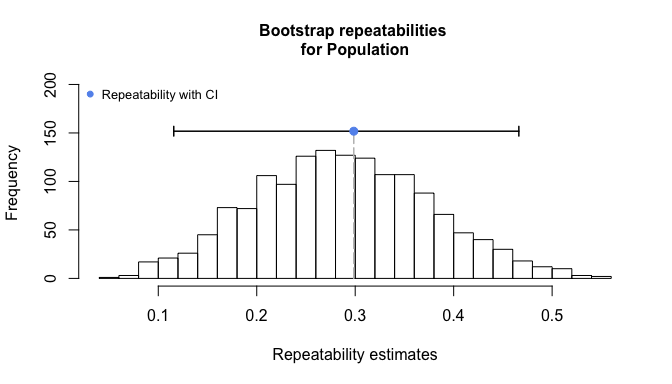Parametric bootstrapping and permutations are inherently repetitive procedures that can be computed on multiple cores in parallel. The rptR package has build-in options for using multiple cores by setting parallel = TRUE. The default ncores = NULL determines the number of cores on your machine and uses all but one core. It is also possible to specify the number of cores to be used via the ncores argument.

The statistical significance of the repeatability is tested by likelihood ratio tests (LRT), comparing the model fit of a model including the grouping factor of interest and one excluding it (i.e., constraining it group-level variance to zero). Because the LRT are conducted at the boundary of possible parameter space (against zero group-level variance), the difference in log-likelihoods is assumed to follow a mixture distribution of a $$\chi^2$$-distribution with 1 degree-of-freedom and point mass at zero. $$P$$ values based on LRT are part of the standard print output and the full details can be see by a call to summary (see above). In this case the variance among populations is highly significant and so is the repeatability at this level.

Permutation can be used as a robust alternative or addition to LRT. In the simplest case this will work by randomizing the vector of group identities against the response vector followed by refitting the model to the randomized data and storage of the repeatability for each of many replications. However, in more complex models involving multiple random effects and/or fixed effects, this will also break the data structure between the grouping factor of interest and other aspects of the experimental design. We therefore construct randomized datasets by fitting a model without the grouping factor of interest and then add the permutated residuals to the fitted values of this model. This maintains the general data structure and any effects of other design aspects on the response while still breaking the link between grouping factor and the response.

rep2 <- rpt(BodyL ~ (1 | Population), grname = "Population", data = BeetlesBody,
datatype = "Gaussian", nboot = 0, npermut = 1000)

It is not unusual to see warnings about convergence problems, because the data are simulated with zero group-level variance and this might occasionally produce odd data. The results of the permutation tests are shown as part of print and summary, and can be visualized using the type argument in the plot function. The results are in general agreement with the LRT in this case, but note that the resolution of the permutation tests is limited by the number of iterations. Since the empirical estimate is always included as one permutation sample (following the reasoning that even under the null hypothesis, the observed data are a possible, albeit possibly unlikely, outcome, Manly 2007), the resolution is limited to $$1/N$$ were $$N$$ is the number of permutations. It is typical to see the spike at zero and an extended right tail if variances are low.

plot(rep2, type = "permut", cex.main = 1)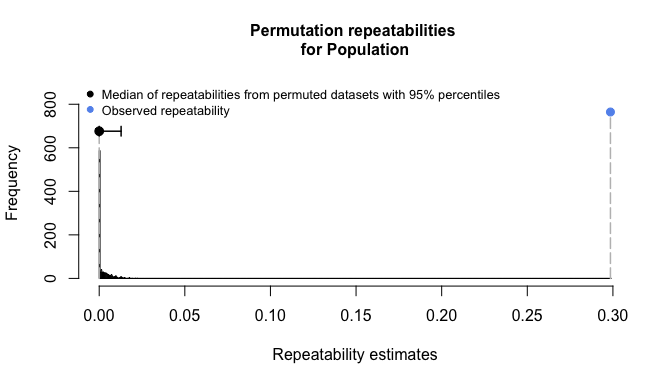The full return object can be viewed by the str functions, which shows, for example, that R_boot holds all parametric bootstrapping samples, R_permut all permutation samples (the first one of which is the point estimate), all_warnings hold all warning messages and mod holds the model fit as an lmerMod as returned by lmer.

str(rep2)
# Output omitted

## Estimating enhanced agreement repeatabilities

It is sometimes desiarable to fit models with fixed effects without loosing the variance explained by fixed effects from the denominator. We have therefore introduced the argument adjusted. It defaults to TRUE, which means that adjusted repeatabilites are estimated. But when the argument is set to FALSE, the function will estimate what we here call the enhanced agreement repeatability, because it allows to fit an improved model without loosing the variance from the repeatability denominator of the repeatability. With adjusted = FALSE the variance explained by fixed effects will be calculated by the variance in the linear predictor (on the link scale for non-Gaussian data). This variance is added to the denominator of the repeatability equation just as other variance components.

rep6 <- rpt(BodyL ~ Treatment + Sex + (1 | Container) + (1 | Population), grname = c("Container",
"Population"), data = BeetlesBody, datatype = "Gaussian", nboot = 1000,
npermut = 0, adjusted = FALSE)
print(rep6)
##
##
## Repeatability estimation using the lmm method
##
## Repeatability for Container
## R  = 0.051
## SE = 0.013
## CI = [0.027, 0.078]
## P  = 1.34e-13 [LRT]
##      NA [Permutation]
##
## Repeatability for Population
## R  = 0.299
## SE = 0.089
## CI = [0.127, 0.47]
## P  = 3.19e-31 [LRT]
##      NA [Permutation]

In our example, it turns out that the fixed effects explain a substantial amount of phenotypic variance and this reduces the relative contribution of Population and Container. We will see below that the change is entirely explained by the fraction of the phenotypic variance explained by fixed effects. Note that the repeatability for Population is now the same as in our initial model (rep1), although a far more complex model was fitted. The estimation of enhanced agreement repeatabilities works equally for the non-Gaussian models introduced below.

## Estimating the coefficient determination $$R^2$$ for fixed effects

If we are able to feed the variance explained by fixed effects to the denominator of the repeatability calculation, it is also possible to extract the variance explained by fixed effects in the model as a quantity of interest. We have introduced a reserved term Fixed to the grname argument for this purpose. In combination with ratio = FALSE, grname = "Fixed" will return the variance in the linear predictor (on the latent scale for non-Gaussian data). In combination with ratio = TRUE, grname = "Fixed" will return the fraction of the total variance that is explained by variance in the linear predictor, i.e. by fixed effects. Incidently, the combination grname = "Fixed", ratio = TRUE estimates a form of the coefficient of determination $$R^2$$ that we have called the marginal $$R^2$$ of mixed effects models (Nakagawa & Schielzeth 2013). rptR provides a quantification of uncertainty for the variance explained by fixed effects by parametric bootstrapping as for other variance components. However, we have not implemented significance testing for the fixed effect variance. This should be quantified by other means.

rep7 <- rpt(BodyL ~ Treatment + Sex + (1 | Container) + (1 | Population), grname = c("Container",
"Population", "Fixed"), data = BeetlesBody, datatype = "Gaussian", nboot = 1000,
npermut = 0, adjusted = FALSE)
print(rep7)
##
##
## Repeatability estimation using the lmm method
##
## Repeatability for Container
## R  = 0.051
## SE = 0.013
## CI = [0.027, 0.08]
## P  = 1.34e-13 [LRT]
##      NA [Permutation]
##
## Repeatability for Population
## R  = 0.299
## SE = 0.091
## CI = [0.12, 0.468]
## P  = 3.19e-31 [LRT]
##      NA [Permutation]
##
## Repeatability for Fixed
## R  = 0.391
## SE = 0.053
## CI = [0.294, 0.5]
## P  = NA [LRT]
##      NA [Permutation]
plot(rep7, grname = "Fixed", type = "boot")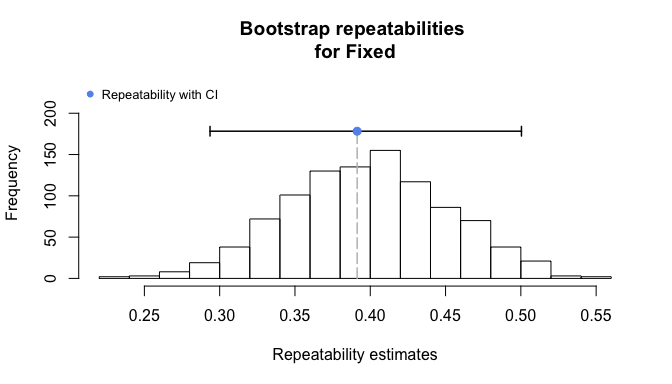The variances explained by container and population are evidently unchanged, but it is now visible that the fixed effects alone explain almost 40% of the phenotypic variance in body length in our example. A big part of this is simply sexual dimorphism. This serves as an example, because in this particular case, we would probably be more interested in the adjusted repeatability controlling for Treatment and Sex.

## Repeatabilities for Poisson-distributed count data

Count data represent a typical situation in which we might want to consider fitting generalized linear mixed-effects models (GLMM). Poisson error distributions with log-link are often appropriate for count data, although there is no guarantee that count data will be best modeled by Poisson error distributions (negative binomial distributions are an obvious alternative). Using the link function, the model parameters are fitted on the latent scale rather than on the original data scale and consequently the model output will represent results on the latent scale.

While in a Gaussian model, there are different parameters for the location and the spread of the distribution (mean and variance), this is not the case in non-Gaussian models. The Poisson distribution, for example, has an inherent variance that is equal to the mean. This inherent variance needs to be respected when quantifying repeatabilities for non-Gaussian data. We have reviewed the literature on non-Gaussian link-scale variances (Nakagawa & Schielzeth 2010) and rptR is based on the formula presented therein. It turns out that the distribution-specific variance for a Poisson model with log link is approximated by $$ln(1/\lambda + 1)$$ where $$\lambda$$ is the average count. This approximation however becomes increasingly inaccurate at low $$\lambda$$.

In order to account for additive overdisperison in Poisson and other non-Gaussian models (except for binary data), the current implementation of rptR internally adds an observation level random effect to the model specified in formula. An observation level random effect is an additional random effect term (named Overdispersion) with as many levels as there are observations to the specified model. The additive overdispersion explained by the new Overdispersion term is added to the distribution-specific variance in the denominator of the repeatability. See als section ‘Multiplicative and additive overdispersion models’ towards the end of the vignette.

As an example, we will analyse the number of eggs laid by female beetles (Eggs) in our toy dataset. We will estimate the repeatability at the level of the population as well as at the level of the container simultaneously while controlling for excess variation introduced by the experimental nutritional treatment. There is no need to control for sex-differences in this sex-limited trait.

data(BeetlesFemale)
hist(BeetlesFemale$Egg, nclass = max(BeetlesFemale$Egg))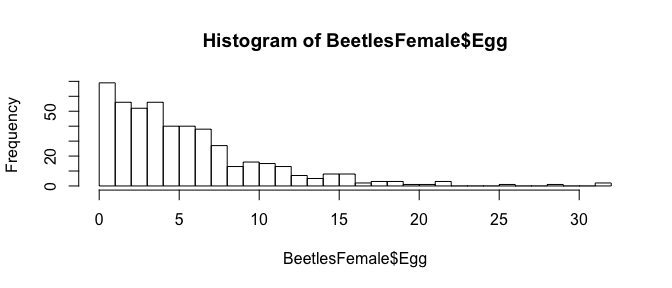We will estimate the repeatability through the rpt function using the datatype = "Poisson" argument. Alternatively, it would be possible to fit the model directly using the rptPoisson function. The formula syntax follows the rules used in glmer from the lme4 package, which is also the central engine for fitting the mixed effects model.

rep8 <- rpt(Egg ~ Treatment + (1 | Container) + (1 | Population), grname = c("Container",
"Population"), data = BeetlesFemale, datatype = "Poisson", nboot = 1000,
npermut = 0)

In non-Gaussian models, it frequently happens that some of the bootstrap or permutation iterations do notF converge on a good model fit. Warnings are thrown by glmer and are collected in the warnings element of the rpt return object.

As usual, the output can be viewed and displayed by print, summary and plot. The results show substantial repeatability at the level of the population (due to genetic differentiation or lasting environmental influence prior to sampling), but very little repeatability at the level of the container (indicating limited shared environmental effects).

print(rep8)
##
##
## Repeatability estimation using the glmm method and log link
##
## Repeatability for Container
## --------------------------------
## Link-scale approximation:
## R  = 0.038
## SE = 0.025
## CI = [0, 0.1]
## P  = 0.00874 [LRT]
##      NA [Permutation]
##
## Original-scale approximation:
## R  = 0.032
## SE = 0.023
## CI = [0, 0.089]
## P  = 0.00874 [LRT]
##      NA [Permutation]
##
## Repeatability for Population
## --------------------------------
## Link-scale approximation:
## R  = 0.52
## SE = 0.122
## CI = [0.234, 0.689]
## P  = 1.19e-16 [LRT]
##      NA [Permutation]
##
## Original-scale approximation:
## R  = 0.504
## SE = 0.121
## CI = [0.223, 0.68]
## P  = 1.19e-16 [LRT]
##      NA [Permutation]

There are two repeatabilities for Poisson GLMM, derived by a latent scale approximation and a original scale approximation. We have previously called the two estimates the latent scale repeatability and the original scale repeatability. However, they are both estimates of the same quantities, not entirely different repeatabilites, hence we now prefer to call them the latent and orginal scale approximations. In the case of Poisson GLMM, original scale approximations are actually the exact solutions, while link scale approximations are indeed approximate. However, in our experience the two typically give very similar results.

plot(rep8, grname = "Container", scale = "link", cex.main = 0.8)
plot(rep8, grname = "Population", scale = "link", cex.main = 0.8)
plot(rep8, grname = "Container", scale = "original", cex.main = 0.8)
plot(rep8, grname = "Population", scale = "original", cex.main = 0.8)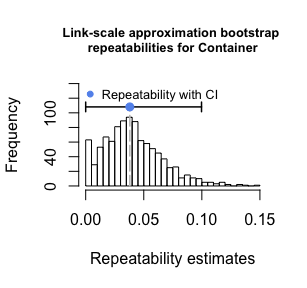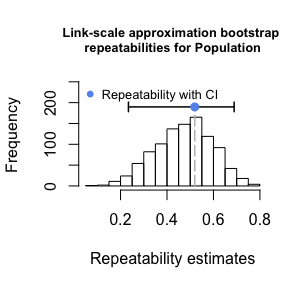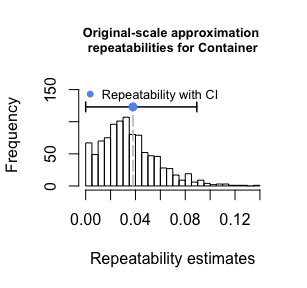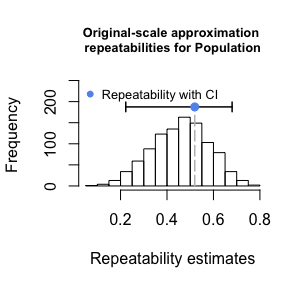As with Gaussian models, it is possible to estimate enhanced agreement repeatabilities as well as the proportion of variances explained by fixed effects (marginal $$R^2$$). In this case the treatment effect explained less than 10% of the variance and including this fraction to the denomionator of the repeatability calculation gives marginally reduced repeatability estimates for population and container:

rep9 <- rpt(Egg ~ Treatment + (1 | Container) + (1 | Population), grname = c("Container",
"Population", "Fixed"), data = BeetlesFemale, datatype = "Poisson", nboot = 1000,
npermut = 0, adjusted = FALSE)
print(rep9)
print(rep9)
##
##
## Repeatability estimation using the glmm method and log link
##
## Repeatability for Container
## --------------------------------
## Link-scale approximation:
## R  = 0.034
## SE = 0.022
## CI = [0, 0.089]
## P  = 0.00874 [LRT]
##      NA [Permutation]
##
## Original-scale approximation:
## R  = 0.022
## SE = 0.015
## CI = [0, 0.059]
## P  = 0.00874 [LRT]
##      NA [Permutation]
##
## Repeatability for Population
## --------------------------------
## Link-scale approximation:
## R  = 0.468
## SE = 0.117
## CI = [0.195, 0.643]
## P  = 1.19e-16 [LRT]
##      NA [Permutation]
##
## Original-scale approximation:
## R  = 0.344
## SE = 0.097
## CI = [0.132, 0.497]
## P  = 1.19e-16 [LRT]
##      NA [Permutation]
##
## Repeatability for Fixed
## --------------------------------
## Link-scale approximation:
## R  = 0.1
## SE = 0.028
## CI = [0.059, 0.164]
## P  = NA [LRT]
##      NA [Permutation]
##
## Original-scale approximation:
## R  = 0.065
## SE = 0.017
## CI = [0.038, 0.104]
## P  = NA [LRT]
##      NA [Permutation]

The quantification of the distribution-specific variance of Poisson models with log-link requires a parameter $$\lambda$$ that represents the expected value, i.e. the global mean on the data scale. We have previously given different advice for estimating $$\lambda$$, including the inverse-link of the intercept on the latent scale. While this gives a reasonable approximation in many cases, the estimate will be biased for small expected values (smaller than about 2). A preferred alternative is to use the mean of the observations in the sample as we have also suggested previously. The is now the default option in rptR that is determined by the expect argument with its default expect = "meanobs".

There is also an analytical alternative that takes the intercept and variances as estimated on the latent scale. The expected value is $$E[y] = exp(\beta_0 + var_{tot}/2)$$, where $$\beta_0$$ represents the intercept on the latent scale and $$var_{tot}$$ represents the sum of the variances, including variance caused by fixed effects, on the latent scale. While this is generally a preferable solution, the estimates are susceptible to the distribution of covariates and, in most cases, it gives appropriate results only if all covariates are centered. Since centering is not enforced within rptR, we have made this an opt-in option that can be used by setting expect = "latent". The repeatabilities estimated by the formulae presented in Nakagawa & Schielzeth (2010) can be requested by expect = "liability".

## Repeatabilities for Binary data

Binary data are sets of single observations with failure/success status (coded 0/1). They are a special case of proportion data (see below) with a sample size of 1 per observation. Because of a number of specificities, repeatabilities for binary and proportion data are separately coded in rptR. For example, binary models to not fit the addional overdispersion term, since there is not definable overdispersion in binary models. Binary data are handed over to rpt as a single vector of 0’s and 1’s or as a two-level factor.

Males in our toy example occur in two distinct color morphs: dark and reddish-brown. We will analyze the repeatability of color morph identities (Colour) as an example for the repeatability of binary data. This is a test for spatial heterogeneity in morph ratios.

data(BeetlesMale)
table(c("dark", "reddish")[BeetlesMale$Colour + 1]) ## ## dark reddish ## 275 205 We estimate the repeatability through the wrapper function rpt at the level of population and at the level of containers. As with Poisson models it frequently happens that some of the bootstrap or permutation iterations do not converge on a model fit and that glmer throws warnings that are collected in the all_warnings element of the rpt object. rep10 <- rpt(Colour ~ (1 | Container) + (1 | Population), grname = c("Container", "Population"), data = BeetlesMale, datatype = "Binary", nboot = 1000, npermut = 0) print(rep10) ## ## ## Repeatability estimation using the glmm method and logit link ## ## Repeatability for Container ## -------------------------------- ## Link-scale approximation: ## R = 0 ## SE = 0.014 ## CI = [0, 0.051] ## P = 1 [LRT] ## NA [Permutation] ## ## Original-scale approximation: ## R = 0 ## SE = 0.014 ## CI = [0, 0.051] ## P = 1 [LRT] ## NA [Permutation] ## ## Repeatability for Population ## -------------------------------- ## Link-scale approximation: ## R = 0.188 ## SE = 0.073 ## CI = [0.042, 0.33] ## P = 5.81e-09 [LRT] ## NA [Permutation] ## ## Original-scale approximation: ## R = 0.185 ## SE = 0.071 ## CI = [0.042, 0.322] ## P = 5.81e-09 [LRT] ## NA [Permutation] As with Poisson GLMM, there are two approximations for the repeatability that we call the latent scale approximation and original scale approximation and in the case of Binomial GLMM, the two are both approximations of the same quantity and in our experience the two typically give very similar results. It turns out that there is significant repeatability at the level of populations, but not on the level of containers (at least not beyond variance among populations). It frequently happens that small variances are estimated as zero, because small variances are difficult to estimate with any level of confidence. For a check of robustness, we still estimate the enhanced agreement repeatabilities controlling for the treatment manipulation, but it turns out that this change to the model does hardly affect the repeatability estimates due to the low explanatory power of the treatment. rep11 <- rpt(Colour ~ Treatment + (1 | Container) + (1 | Population), grname = c("Container", "Population", "Fixed"), data = BeetlesMale, datatype = "Binary", nboot = 1000, npermut = 0, adjusted = FALSE) print(rep11) ## ## ## Repeatability estimation using the glmm method and logit link ## ## Repeatability for Container ## -------------------------------- ## Link-scale approximation: ## R = 0 ## SE = 0.014 ## CI = [0, 0.05] ## P = 0.5 [LRT] ## NA [Permutation] ## ## Original-scale approximation: ## R = 0 ## SE = 0.013 ## CI = [0, 0.046] ## P = 0.5 [LRT] ## NA [Permutation] ## ## Repeatability for Population ## -------------------------------- ## Link-scale approximation: ## R = 0.196 ## SE = 0.074 ## CI = [0.054, 0.339] ## P = 5.55e-09 [LRT] ## NA [Permutation] ## ## Original-scale approximation: ## R = 0.174 ## SE = 0.059 ## CI = [0.052, 0.276] ## P = 5.55e-09 [LRT] ## NA [Permutation] ## ## Repeatability for Fixed ## -------------------------------- ## Link-scale approximation: ## R = 0.045 ## SE = 0.019 ## CI = [0.016, 0.09] ## P = NA [LRT] ## NA [Permutation] ## ## Original-scale approximation: ## R = 0.04 ## SE = 0.016 ## CI = [0.015, 0.076] ## P = NA [LRT] ## NA [Permutation] plot(rep11, grname = "Population", scale = "link", cex.main = 0.8) plot(rep11, grname = "Population", scale = "original", cex.main = 0.8) plot(rep11, grname = "Container", scale = "link", cex.main = 0.8) plot(rep11, grname = "Container", scale = "original", cex.main = 0.8) plot(rep11, grname = "Fixed", scale = "link", cex.main = 0.8) plot(rep11, grname = "Fixed", scale = "original", cex.main = 0.8)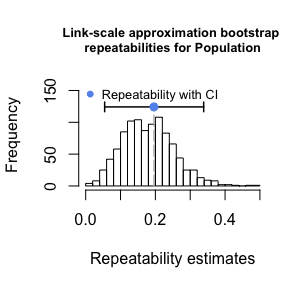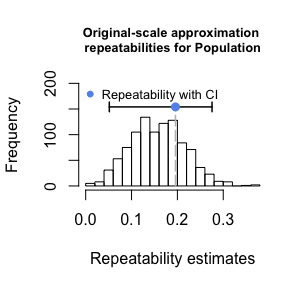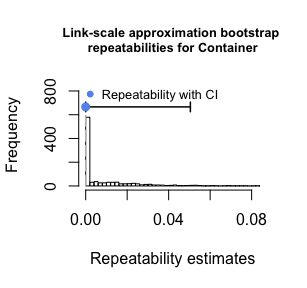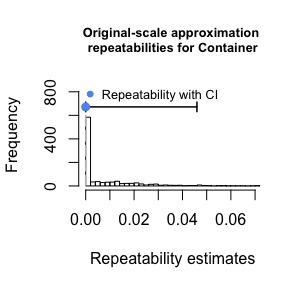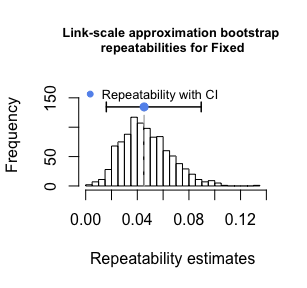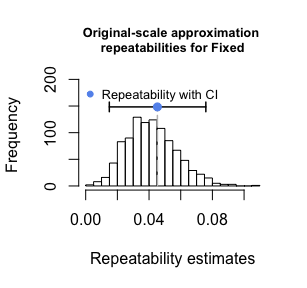We have previously adviced to estimate the distribution specific variance for the link scale approximation as $$\pi^2/3$$ (Nakagawa & Schielzeth 2010). While this gives a reasonable approximation, it is preferable to estimate the link scale variance as $$1 / (p(1-p))$$ where $$p$$ is the expected probability of success (Nakagawa & Schielzeth 2016). The current version of rptR implements the more accurate approximation $$1 / (p(1-p))$$. As with Poisson GLMM, we have implemented two options for estimating the expectation for $$p$$. The default version with expect = "meanobs" takes the average succes rate across all observations. There is also a latent scale approximation (details are presented in Nakagawa & Schielzeth 2016) that can be used upon choice by setting expect = "latent". As with Poisson GLMM, we consider the analytical solution via expect = "latent" more accurate and putitatively more robust to unbalanced sampling, but expect = "meanobs" seems safer, because it does not depend on how covariates are coded. ## Repeatabilities for Proportion data Counts of successes and failures are conveniently modelled as proportion data. They are handed over to rpt (and ultimately to glmer) as combined vectors of integers. We will illustrate the repeatability analysis of proportion data by aggregating color morph identities within containers. The analysis is equivalent to the analysis of the data as binary data with Container treated as a random effect as it was done above (model rep10). BeetlesMale$Dark = BeetlesMale$Colour BeetlesMale$Reddish = (BeetlesMale\$Colour - 1) * -1
BeetlesMaleAggr <- aggregate(cbind(Dark, Reddish) ~ Population + Container,
data = BeetlesMale, FUN = sum)
rep12 <- rpt(cbind(Dark, Reddish) ~ (1 | Population), grname = c("Population"),
data = BeetlesMaleAggr, datatype = "Proportion", nboot = 1000, npermut = 0)
print(rep12)
print(rep12)
##
##
## Repeatability estimation using the glmm method and logit link
##
## Repeatability for Population
## --------------------------------
## Link-scale approximation:
## R  = 0.188
## SE = 0.072
## CI = [0.045, 0.318]
## P  = 5.81e-09 [LRT]
##      NA [Permutation]
##
## Original-scale approximation:
## R  = 0.185
## SE = 0.07
## CI = [0.045, 0.308]
## P  = 5.81e-09 [LRT]
##      NA [Permutation]

## Multiplicative and additive overdispersion models

There are two alternative ways of modelling overdispersion in Poisson and proportion models. In the current implementation of rptR we use the underlying glmer model fits for estimating additive overdispersion as excess variation at the latent level (excess beyond variability arising from the sampling distribution alone). It is implemented as an additional random effect term (named Overdispersion) with as many levels as there are observations. This variation is added to the distribution-specific variance in the denominator of the repeatability.

Multiplicative overdispersion, on the contrary, models overdispersion as a parameter (conveniently labelled $$\omega$$) that multiplies the distribution-specific variance. $$\omega = 1$$ is thus equivalent to no overdispersion beyond what is expected from the distribution. See Nakagawa & Schielzeth (2010) for details on additive and multiplicative overdispersion.

The choice of additive versus multiplicative overdispersion is a matter of convenience. The data for the toy example was simulated as additive overdispersion and it happens that the powerful glmer function implements additive overdispersion. We have previously used glmmPQL for fitting multiplicative overdispersion models, but do not maintain this option any longer.

## Estimating variances instead of ratios

We wrote rptR with the focus primarily on estimating repeatabilities, including a quantification of their uncertainty. However, we realize that it is often of interest to estimate variances and their uncertainty directly rather than as ratios of variances. rptR can be used for this purpose too, by setting the ratio argument from TRUE (the default) to FALSE.

For example, if we are interested in the link scale variances of colour morph variation, we can estimate the variances as:

rep13 <- rpt(Egg ~ Treatment + (1 | Container) + (1 | Population), grname = c("Container",
"Population"), data = BeetlesFemale, datatype = "Poisson", nboot = 1000,
npermut = 0, ratio = FALSE)
print(rep13)
##
##
## Variance estimation using the glmm method and log link
##
## Variance of Container
## --------------------------------
## Link-scale approximation:
## Var = 0.022
## SE = 0.012
## CI = [0.001, 0.05]
## P  = 0.00874 [LRT]
##      NA [Permutation]
##
## Variance of Population
## --------------------------------
## Link-scale approximation:
## Var = 0.304
## SE = 0.126
## CI = [0.09, 0.605]
## P  = 1.19e-16 [LRT]
##      NA [Permutation]
plot(rep13, grname = "Container", scale = "link", cex.main = 0.8)
plot(rep13, grname = "Population", scale = "link", cex.main = 0.8)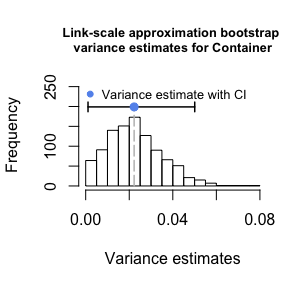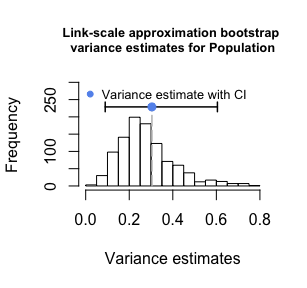Evidently, the significance of the random effect terms won’t change no matter whether they are presented as variances or as variances devided by the phenotypic variance (repeatabilities). If we are interested in inspecting raw variances, we are often also interested in quantifying the amount of residual variance and its uncertainty, too. We have therefore introduced two addition reserved terms for the grname argument (besides grname = "Fixed") to allow this. Residual will allow estimating the residual variance, which in the case of non-Gaussian models is the overdispersion on the latent scale plus the distribution-specific variance. Overdispersion will allow estimating the overdispersion variance and the latent scale, which in the case of Gaussian models (with implicit identity link) is equal to the residual estimated by Residual.

It is necessary to specify at least one additional grouping level in the grname argument along with Residual. The results for Container and Population are unaffected and are the same as above (rep13). In our example there is significant overdispersion, meaning that besides treatment effects and variation among populations and among containers within populations there is more unexplained variation than expected from the Poisson distribution alone.

rep14 <- rpt(Egg ~ Treatment + (1 | Container) + (1 | Population), grname = c("Container",
"Population", "Overdispersion", "Residual"), data = BeetlesFemale, datatype = "Poisson",
nboot = 1000, npermut = 0, ratio = FALSE)
print(rep14)
##
##
## Variance estimation using the glmm method and log link
##
## Variance of Container
## --------------------------------
## Link-scale approximation:
## Var = 0.022
## SE = 0.012
## CI = [0.002, 0.05]
## P  = 0.00874 [LRT]
##      NA [Permutation]
##
## Variance of Population
## --------------------------------
## Link-scale approximation:
## Var = 0.304
## SE = 0.133
## CI = [0.084, 0.582]
## P  = 1.19e-16 [LRT]
##      NA [Permutation]
##
## Variance of Overdispersion
## --------------------------------
## Link-scale approximation:
## Var = 0.103
## SE = 0.019
## CI = [0.062, 0.138]
## P  = 2.22e-17 [LRT]
##      NA [Permutation]
##
## Variance of Residual
## --------------------------------
## Link-scale approximation:
## Var = 0.259
## SE = 0.032
## CI = [0.202, 0.328]
## P  = NA [LRT]
##      NA [Permutation]
plot(rep14, grname = "Overdispersion", scale = "link", cex.main = 0.8)
plot(rep14, grname = "Residual", scale = "link", cex.main = 0.8)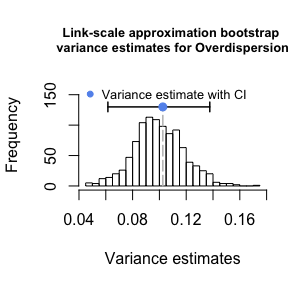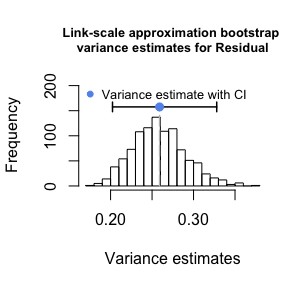It is pointless to test for the statistical significance of residual variance against a null hypothesis of no residual variance and we therefore omit this test. But for non-Gaussian models it is worthwhile testing if there is statistically significant overdispersion and we have therefore implemented the LRT test (but not the permutation test) for Overdispersion. In gerneral, however, be aware that each grouping level specified in grname will be tested in separate iterations if a permutation test is required. Hence it is recommened to be careful with the use of npermut in combination with multiple grouping levels.

rep15 <- rpt(Egg ~ Treatment + (1 | Container) + (1 | Population), grname = c("Container",
"Population", "Overdispersion"), data = BeetlesFemale, datatype = "Poisson",
nboot = 0, npermut = 1000, ratio = FALSE)
print(rep15)
##
##
## Variance estimation using the glmm method and log link
##
## Variance of Container
## --------------------------------
## Link-scale approximation:
## Var = 0.022
## SE = NA
## CI = [NA, NA]
## P  = 0.00874 [LRT]
##      0.211 [Permutation]
##
## Variance of Population
## --------------------------------
## Link-scale approximation:
## Var = 0.304
## SE = NA
## CI = [NA, NA]
## P  = 1.19e-16 [LRT]
##      0.088 [Permutation]
##
## Variance of Overdispersion
## --------------------------------
## Link-scale approximation:
## Var = 0.103
## SE = NA
## CI = [NA, NA]
## P  = 2.22e-17 [LRT]
##      NA [Permutation]

It is possible to use both Overdisperion and Residual in combination with ratio = FALSE or with ratio = TRUE. The ratio of the residual variance to the total variance should then be interpreted as the ‘non-repeatability’. We assume that the combination of these two terms with ratio = FALSE will be more commonly used.

## Random-slope models

It is possible to fit random-slope models following the approach introduced by Johnson (2014). The function call will return the average repeatability across the distribution of the covariate in the sample (see Johnson 2014 for details). Permutation tests and likelihood ratio test are conducted with random-intercept and random-slope terms being jointly removed from the model. Note that random-slope models can be specified in multiple ways and the function will likely handle only those models well that have been specified in the classical way (foo | grname), implicitly fitting random-intercepts, random slopes for the covariate foo and their correlation. Multiple random-slopes are possible, but models with covariances being constraint do currently not work.

The following code gives and example for a repeatability estimation for body size where random slopes are fitted for Sex and Treatment at the level of populations. The resulting model allows for variance components to vary by sex and by treatment with the average across the sex/treatment levels being returned as the model output.

rep16 <- rpt(BodyL ~ Treatment + Sex + (1 | Container) + (Treatment + Sex |
Population), grname = c("Population"), data = BeetlesBody, datatype = "Gaussian",
nboot = 500, npermut = 0, adjusted = FALSE)
summary(rep16)
##
## Repeatability estimation using the lmm method
##
## Call = rpt(formula = BodyL ~ Treatment + Sex + (1 | Container) + (Treatment + Sex | Population), grname = c("Population"), data = BeetlesBody, datatype = "Gaussian", nboot = 500, npermut = 0, adjusted = FALSE)
##
## Data: 960 observations
## ----------------------------------------
##
## Population (12 groups)
##
## Repeatability estimation overview:
##       R     SE   2.5%  97.5% P_permut  LRT_P
##   0.303 0.0854  0.129  0.473       NA      0
##
## Bootstrapping and Permutation test:
##             N   Mean Median   2.5%  97.5%
## boot      500  0.293  0.294  0.129  0.473
## permut      1     NA     NA     NA     NA
##
## Likelihood ratio test:
## logLik full model = -1526.295
## logLik red. model = -1595.399
## D  = 138, df = 6, P = 2.39e-27
##
## ----------------------------------------

## Concluding remarks

The rptR package offers convenient extraction of (ratios of) variance components for multiple grouping levels for estimating repeatabilities, including the option to control for fixed effects in order to estimate adjusted repeatabilities. While this is easy-to-use we want to remind users to make sure their data is appropriately modeled. We therefore provide the fitted mixed model as part of the rpt object and recommend that this is inspected for any indications of violated model assumptions or missing predictors. Repeatability estimates from Poisson, binary and proportion data might often appear disappointingly low. This is likely to be a simple reflection of the fact that for non-Gaussian data, the numerator contains the among-group variance in predispositions (e.g. probabilities of successes, long-range averages of counts) while the observed data is discretely scattered around these expectations. A lot of the phenotypic variance is hence inherently random in origin and each datapoint contains only very limited information about the underlying predisposition. The estimation of repeatabilities for count, binary and proportion data will thus require substantial sample sizes and (ideally) optimized sampling designs.